Next: Other Constructions Up: SUPERSTRING PHENOMENOLOGY BEFORE 1995 Previous: Orbifold Compactifications

## Calabi-Yau compactifications

We saw that the orbifolds obtained from twisting the 6D tori can give rise to chiral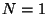models in 4D. Orbifolds are singular objects but they can be smoothed out by blowing-up the singularities at the fixed points. The resulting smooth manifold is a so-called Calabi-Yau manifold . Mathematically, these are 6D complex manifolds with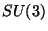holonomy or equivalently vanishing first Chern class. They were actually the first standard Kaluza-Klein compactification considered in string theory, leading to chiral 4D models and generically gauge group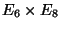, with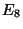a hidden gauge group.

The drawback of compactifications on Calabi Yau manifolds is that they are highly nontrivial spaces and we cannot describe the strings on such manifolds, contrary to what we did in the case of free theories such as tori and orbifolds. In particular we can not compute explicitly the couplings in the effective theory, except for the simplest renormalizable Yukawa couplings.

On the other hand, Calabi-Yau manifolds have been understood much better during the past few years and have lead to some beautiful and impressive results. In a way they are more general than orbifolds because an orbifold is only a particular singular limit of a Calabi-Yau manifold. Also there are other constructions of these manifolds which are not related to orbifolds. They can be defined as hypersurfaces in complex (weighted) projective spaces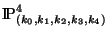where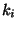's are the weights of the corresponding coordinates for which there is the identification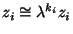. The hypersurface is defined as the vanishing locus of a polynomial of the corresponding coordinates. For instance the surface defined as: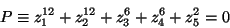(13)

defines a Calabi-Yau manifold with weights: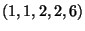. The relation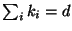where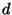is the degree of the polynomial ensures that the surface is a Calabi-Yau manifold. The manifold is guaranteed to be smooth if both the polynomial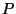and its derivatives do not vanish simultaneously. Larger classes of manifolds can be constructed by considering intersections of hypersurfaces in higher dimensional projective spaces, the so-called complete intersection Calabi-Yau manifolds (CICY). Actually, it is known in the mathematical literature, that all Calabi-Yau spaces can be defined as (intersection of) hypersurfaces in weighted projective spaces. Large numbers of these manifolds have been classified, although the full classification is not complete. The mathematical estimate is that there are of the order of ten thousand Calabi-Yau manifolds. The corresponding string models are of the type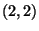. Some of the highlights of Calabi-Yau compactifications are the following:

(i) There are classes of moduli fields, generalizing the fields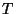and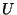of the two-torus mentioned before. The number of these fields is given by topological numbers known as Hodge numbers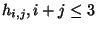. They correspond to the number of complex harmonic forms that can be defined in the manifold with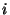holomorphic indices and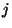antiholomorphic indices. Then the number of complex structure fields (U) is given by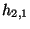and the number of Kähler structure fields () is given by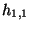. Many of theforms correspond to the coefficients of different monomials which can be added to the defining polynomial that still give rise to the same space, other forms correspond to the blowing up of possible singularities. Many of theforms correspond to polynomial deformations of the defining surface, but others are related with the reparation of singularities. For Calabi-Yau manifolds we have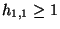, therefore there is always a special Kähler class deformation which can be thought as the overall size of the corresponding manifold, it is usually called, the Kähler form. All the other Hodge numbers of Calabi-Yau manifolds are fixed (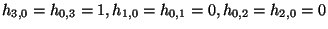)

(ii) The gauge group in 4D is. The matter fields transform as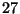's or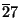's of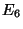. The number of each is also given by the Hodge numbers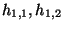and the number of generations is then topological: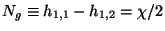where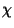is the Euler number of the manifold. This is one of the most appealing properties of these compactifications since they imply that topology determines the number of quarks and leptons.

(iii)Mirror symmetry . Similar to the 2D toroidal compactifications, it has been found that there is a mirror symmetry in Calabi-Yau spaces that exchanges the moduli fields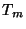and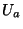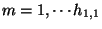,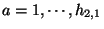. This means that for every Calabi-Yau manifold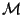, there exists another manifold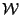which has the complex and Kähler structure fields exchanged, ie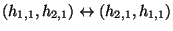and opposite Euler number. The mirror symmetry of the two-torus described previously, is only a special example on which the manifold is its own mirror. Mirror symmetry is not only a nontrivial contribution of string theory to modern mathematics, but it has very interesting applications for computing effective Lagrangians as we will see in the next section. It also relates the geometrical modular symmetries associated to thefields of the manifoldto generalized, stringy,-duality symmetries for the mirrorand viceversa (see for instance  ).

(iv) Even though these string models are not completely understood in terms of 2D CFTs, special points in the moduli space of a given Calabi-Yau are CFTs, such as the orbifold compactifications mentioned before. In fact some people believe that there is a one to one correspondence between string models withsupersymmetry in the worldsheet and Calabi-Yau manifolds. There is also a description of CFTs in terms of effective Landau-Ginzburg Lagrangians which are intimately related to Calabi-Yau compactifications as two phases of the same 2D theory . In particular the potential of that Landau-Ginzburg theory is determined by the polynomialdefining the Calabi-Yau hypersurface.

(v) There are also few models with three generations that, after thesymmetry breaking, could lead to quasi-realistic 4D strings. One of these models was analyzed in some detail . Usually,models also lead to the existence of extra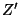particles of different kinds. They have been thoroughly studied because of the potential experimental importance of detecting an extra massive gauge boson (for a recent discussion see ref.  ).

(vi) Although most of the Calabi-Yau models studied so far correspond to standard embedding in the gauge degrees of freedom (models), there is also the possibility of constructing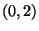models by performing different embeddings, similar to the orbifold case. This increases substantially the number of string models of this construction .Next: Other Constructions Up: SUPERSTRING PHENOMENOLOGY BEFORE 1995 Previous: Orbifold Compactifications
root 2001-01-22